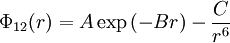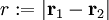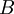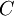# Buckingham potential

Jump to: navigation, search

The Buckingham potential is given by$\Phi_{12}(r) = A \exp \left(-Br\right) - \frac{C}{r^6}$

where$\Phi_{12}(r)$ is the intermolecular pair potential,$r := |\mathbf{r}_1 - \mathbf{r}_2|$, and$A$,$B$ and$C$ are constants.

The Buckingham potential describes the exchange repulsion, which originates from the Pauli exclusion principle, by a more realistic exponential function of distance, in contrast to the inverse twelfth power used by the Lennard-Jones potential. However, since the Buckingham potential remains finite even at very small distances, it runs the risk of an un-physical "Buckingham catastrophe" at short range when used in simulations of charged systems. This occurs when the electrostatic attraction artificially overcomes the repulsive barrier. The Lennard-Jones potential is also about 4 times quicker to compute  and so is more frequently used in computer simulations.